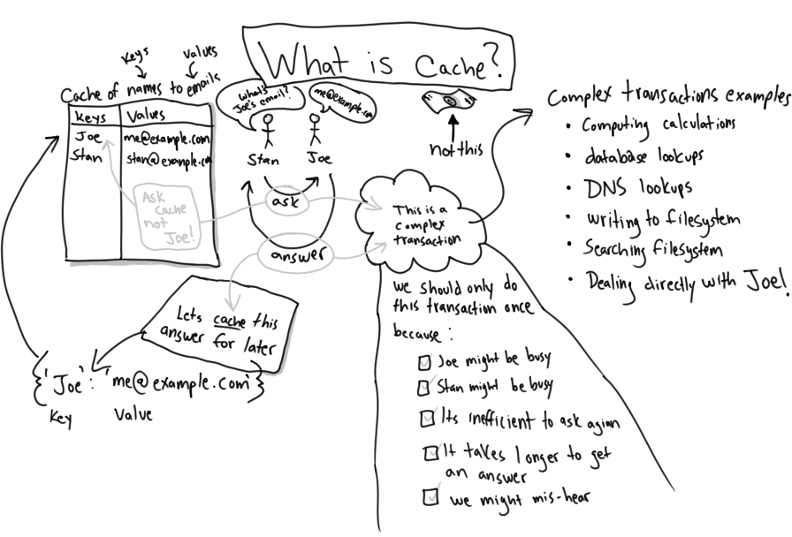Random   •   Archives   •   RSS   •   About   •   Contact

# Explaining cache with python

What is cache? I define cache as "a saved answer to a question". Caching can speed up an application if a computationally complex question is asked frequently. Instead of the computing the answer over and over, we can use the previously cached answer. This post will present one method of adding cache to a python program. Specifically we will write a program that computes prime numbers and saves the answers into cache for quick retrieval.

EDIT: The kind people of the Internet have expressed concern with my loose use of the term cache; the techniques that follow are most accurately described as memoization.

One algorithm for determining if a number is prime follows:

```prime_flag = True # default state
x = 5 # number to test
if x == 1:
prime_flag = False
else:
for i in range( 2, x ):
if x % i == 0:
prime_flag = False
break
print prime_flag
```

Create a prime_flag variable to hold the answer and default it to true. Let x be the number being tested and if x is equal to 1, the x is not prime. Otherwise iterate over each number in the range of 2 to x. Let i be the current number to be tested. if x is divided by i without any remainder, x is not prime. Set the prime_flag to False and break out of the loop. Print the result.

Next we will move the algorithm into a function which will allow for code reuse:

```def is_prime( x ):
"""Determine if a number is prime, return Boolean"""
prime_flag = True
if x == 1:
prime_flag = False
else:
for i in range( 2, x ):
if x % i == 0:
prime_flag = False
break
return prime_flag

# invoke function:
print is_prime( 5 ) # True
print is_prime( 4 ) # False
```

This function saves us a lot of typing and enables the ability to quickly determine if a given number is prime.

Next we will use a python dictionary to implement a result cache. Also by circumstance we introduce objects and classes.

```class Primer( object ):
def __init__( self ):
"""create a cache dictionary"""
self.cache = {}

def is_prime( self, x ):
"""Determine if x is prime, cache and return result"""
if x in self.cache:
return self.cache[x] # lookup result

prime_flag = True

if x == 1:
prime_flag = False
else:
for i in range( 2, x ):
if x % i == 0:
prime_flag = False
break

self.cache[x] = prime_flag # cache result
return prime_flag

p = Primer() # create a new primer object
p.is_prime( 5 ) # True
p.is_prime( 4 ) # False
p.is_prime( 5 ) # True and fetched from cache
```

What is great about this solution is that we can avoid looping and computation if an answer is already in cache. Looking up a cached result is much more efficient and will ultimately make a program feel more responsive.

Determining if 97352613 is prime takes my laptop nearly 18 seconds. Fetching the cached result seems to happen instantly.

```>>> s1 = time();p.is_prime( 97352613 );e1 = time()
False # not prime
>>> s2 = time();p.is_prime( 97352613 );e2 = time()
False # not prime from cache
>>> e1 - s1
17.970067977905273 # seconds
>>> e2 - s2
2.5987625122070312e-05 # or approx .000026 seconds
```

A look-up will always beat a computation. Anything that can be cached, should be cached. I hope this helps clear things up.